# 2nd PUC Basic Maths Previous Year Question Paper March 2019

Students can Download 2nd PUC Basic Maths Previous Year Question Paper March 2019, Karnataka 2nd PUC Maths Model Question Papers with Answers helps you to revise the complete Karnataka State Board Syllabus and score more marks in your examinations.

## Karnataka 2nd PUC Basic Maths Previous Year Question Paper March 2019

Time: 3 Hours 15 Min.
Max.Markss: 100

Instructions:

• Write SI Nos of question correctly
• Visually challenged students need to answer Question No 31(B) instead of map Question No 31(A) in Part D
• Answer the questions according to the instructions given for the questions

Part A

Answer all the Questions: (10 × 1 = 10)

Question 1.
If A = $$\left[ \begin{matrix} 1 & -2 \\ 3 & 4 \end{matrix} \right]$$ then find 2A’.
2A’ = $$\left[ \begin{matrix} 2 & 6 \\ -4 & 8 \end{matrix} \right]$$

Question 2.
In how many ways can 10 people be seated around a table?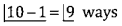Question 3.
Symbolise the proposition:
“If Oxygen is a gas then gold is a compound”.
p → 9Question 4.
Find the triplicate ratio of 5 : 4.
53 : 43 or 125 : 64

Question 5.
Find the income obtained by investing ₹ 3,600 in 5% stock at 90.
Income = $$\frac{5 \times 3600}{90}$$ = ₹200

Question 6.
Express sin 5A cos 3A as sum of difference of two trigonometric functions.
$$\frac { 1 }{ 2 }$$[sin8A + sin 2A]

Question 7.
Find the centre of the circle x2 + y2 – 4xy – 5 = 0.
$$\left(2,+\frac{1}{2}\right)$$

Question 8.
Evaluate : $$\lim _{x \rightarrow 3}\left(\frac{x^{2}-4 x}{x-2}\right)$$
-3

Question 9.
Differentiate (5ex – logx – 3√x) with respect to x.
$$5 e^{x}-\frac{1}{x}-\frac{3}{2 \sqrt{x}}$$

Question 10.
Integrate (x2 – $$\frac { 6 }{ x }$$ + 5ex) with respect to x.
$$\frac{x^{3}}{3} 6 \log _{e} x+5 e^{x}+C$$Part- B

Answer any ten of the following Questions. (10 × 2 = 20)

Question 11.
If A = $$\left[ \begin{matrix} 1 & 3 & -1 \\ -1 & 0 & 2 \end{matrix} \right]$$ and B = $$\left[ \begin{matrix} 4 & -1 & 2 \\ 1 & 3 & -2 \end{matrix} \right]$$
3B = $$\left[ \begin{matrix} 12 & -3 & 6 \\ -4 & -9 & 8 \end{matrix} \right]$$
∴ A = 3B = $$\left[ \begin{matrix} -11 & 6 & -7 \\ -4 & -9 & 8 \end{matrix} \right]$$

Question 12.
In how many ways the word “CARROM” be arranged such that the 2R’s are always together?
₹ 2 as 1 unit
∴ No. of letters = 6 – 2 + 1 = 5Question 13.
A box contains 8 red marbles 6 green marbles and 10 pink marbles. One marble in drawn at random from the box. What is the probability that the marble drawn is either red or green?
Writing 8R + 6G + 10P = 24 OR No. of R & G = 14
∴ Probability = $$\frac{14}{24}=\frac{7}{12}$$

Question 14.
If the truth values of the propositions p,q,r are T,T,F respectively, then find the truth values of p → (q r).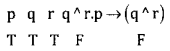Question 15.
What must be added to each term in the ratio 5 : 6 so that it becomes 8 : 9?
$$\therefore \quad \frac{5+x}{6+x}=\frac{8}{9}$$
Solving to get x = 3

Question 16.
True discount on a bill was ₹ 900 and Banker’s gain was ₹ 27. What is the face value of the bill?
Getting BD = ₹ 927
₹ F = Rs. 30,900

Question 17.
Find the value of cos 15°.
cos 15° = cos (45° – 30°)
= cos 45°. cos30° + sin 45°. sin 30°
Getting answer = $$\frac{\sqrt{3}+1}{2 \sqrt{2}}$$Question 18.
If tan A = $$\frac { 1 }{ 2 }$$, tan B = $$\frac { 1 }{ 3 }$$, then find tan (A + B).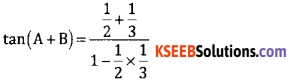Simplifying to get answer = 1

Question 19.
Find the equation of parabola whose vertex is (0,0) and focus is (3,0).
Identifying y2 = 4ax
Getting a = 3 and hence y2 = 12x

Question 20.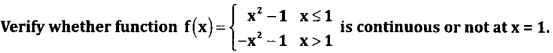Getting LHL = 0 and RHL = -2
Concluding f(x) is not continuous at x = 1.

Question 21.
If y = (a2 – x2)10, find $$\frac{d y}{d x}$$
$$\frac{d y}{d x}$$ = 10(a2 – x2)9(0 – 2x)
= – 20x(a2 – x2)9

Question 22.
If the cost function of a firm is given by C(x) = x3 – 3x + 7. Find the average cost and marginal cost.
AC = x2 – 3 + $$\frac{7}{x}$$
MC = 3x2 – 3

Question 23.
Evaluate : $$\int \frac{4 x+3}{2 x^{2}+3 x+5} d x$$
Substituting 2x2 + 3x + 5 = t
∴ (4x + 3)dx = dt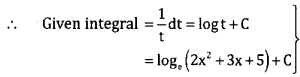Question 24.
Evaluate : $$\int_{1}^{2} x e^{4} d x$$
Writing $$\left[x e^{x}\right]_{1}^{2}-\int_{1}^{2} e^{x} \cdot 1 d x$$
Simplifying to get answer = e2.Part-C

Answer any ten of the following questions: (10 × 3 = 30)

Question 25.
If A = $$\left[ \begin{matrix} 2 & 3 \\ -4 & 1 \end{matrix} \right]$$ and B = $$\left[ \begin{matrix} -1 & 5 \\ 6 & 2 \end{matrix} \right]$$Question 26.
Prove that: $$\left| \begin{matrix} 1+a & b & c \\ a & 1+b & c \\ a & b & 1+c \end{matrix} \right|$$ = 1 + a + b + c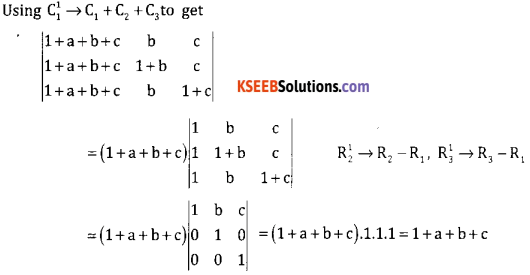Question 27.
From a class of 9 boys and 7 girls 12 students are to be chosen for a competition which includes atleast 6 boys and atleast 4 girls. In how many ways can this be done if a particular boys is always chosen?
No. of ways
= 8C5 × 7C6 + 8C6 × 7C5 + 8C7 × 7C4
= 56 × 7 + 28 × 21 + 8 × 35 = 1260

Question 28.
A couple appears in an interview for two vacancies in the same post. The probability of husband getting selected is $$\frac { 1 }{ 7 }$$ and the probability of wife getting selected is $$\frac { 1 }{ 5 }$$ . What is the probability that
a. Both of them will be selected?
b. Only one of them will be selected?
c. None of them will be selected?
Writing:
P(husband not selected) = $$\frac { 6 }{ 7 }$$  and
P(Wife not selected) = $$\frac { 4 }{ 5 }$$
(OR) Getting:
a. P(Both slected) = $$\frac { 1 }{ 35 }$$
b. P(Only one selected) = $$\frac { 10 }{ 35 }$$ = $$\frac { 2 }{ 7 }$$
C. P(None selected) = $$\frac { 24 }{ 35 }$$Question 29.
In a fort, there was ration for 560 soldiers that would last the soldiers for 70 days after 20 days, 60 soldiers left the fort. For how many days the remaining ration can support the remaining soldiers?
Writing: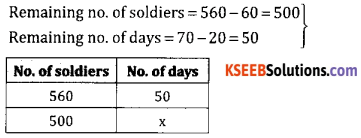Writing:
560 : 500 = x : 50
⇒ x = 56 days

Question 30.
For 512.50 due 6 months at 15% p.a. Find the true present value and discounted cash value.
P = $$\frac{F}{1+t r}$$ = ₹ 7476.74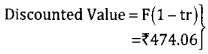OR
Getting P = ₹ 476.74
Getting BD = F.tr = ₹ 38.43Question 31.
Sanjana invests 7 3240 in a stock at 108 and sells when price falls to 104. How much stock at 130 can Sanjana now by?
Case 1: (Buying) stock at 108
Worth of stock purchased = $$\frac{100 \times 3240}{108}$$
= ₹ 3000

Case 2: Stock sold at 104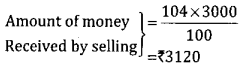Case 3: Stock sold at 130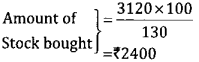Question 32.
Sanju goes to a shop to buy a bicycle quoted at ₹ 2,000. The rate of sales tax is 12% on it. He asks the shopkeeper for a rebate on the price of the bicycle to such an extent that he has to pay ₹ 2016 inclusive of sales tax. Find the rebate percentage on the price of the bicycle.
SP = 2016, ST = 12% Let MP = X
SP = MP + 12% MP
∴ 2016 = x + 0.12%
⇒ x = 1800
⇒ Rebate = 2000 – 1800 = ₹ 200
Rebate $$\%=\frac{200}{2000} \times 100=10 \%$$Question 33.
Find the equation of parabola when vertex is (0,0) and axis is y-axis and passes through the point(-1,-3)
Identifying x2 = 4ay [or x2 = – 4ay]
Substituting x = -1, y = -3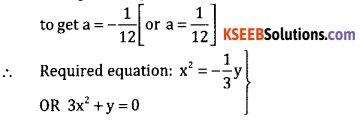Question 34.
Differentiate y = $$\frac{e^{x}-1}{e^{x}+1}$$ with respect to x.
$$\frac{d y}{d x}=\frac{\left(e^{x}+1\right) e^{x}-\left(e^{x}-1\right)\left(e^{x}\right)}{\left(e^{x}+1\right)^{2}}$$
Substituting x = -1, y=-3.

Question 35.
Find the maximum and minimum value of y = x3 – 9x2 + 15 x – 1.
For max or min.
$$\frac{d y}{d x}$$ = 0 ⇒ 3x2 – 18x + 5 = 0
Getting x = 1, x = 5
$$\frac{d^{2} y}{d x^{2}}$$ 6x – 18
At x = 1, $$\frac{d^{2} y}{d x^{2}}$$ = -12
∴ y has max at x = 1
⇒ ymin = 6
At x = 5, $$\frac{d^{2} y}{d x^{2}}$$ = 12
∴ y has min at x = 5
⇒ ymin = -26

Question 36.
A square plate is expanding uniformly, the side is increasing at the rate of 5cm / sec what is the rate at which the area and its perimeter is increasing when the side is 20 cm long?
Area, A = l2 ⇒ $$\frac{\mathrm{d} \mathrm{A}}{\mathrm{dt}}$$ = 2l × $$\frac{\mathrm{d} l}{\mathrm{dt}}$$
= 2 × 20 × 5
$$\frac{\mathrm{d} \mathrm{A}}{\mathrm{dt}}$$ = 200cm2 / sec.
P = 41 ⇒ $$\frac{\mathrm{d} \mathrm{P}}{\mathrm{dt}}$$ = 4 $$\frac{\mathrm{d} l}{\mathrm{dt}}$$ 4 × 5= 20cm / secQuestion 37.
Integrate x2 sin x with respect to x.
∫x sin xdx = -x2 cosx – ∫ -cosx.2xdx
= -x2 cosx + 2[xsinx – ∫sinx.dx]
= -x2 cosx + 2xsinx + 2cosx + C

Question 38.
Evaluate: ∫(2x + 3)(x2 + 3x + 5)3/2 dx.
Substituting x2 – 3x + 5 = t to get (2x + 3) dx = dt
∴ Given integral becomes ∫t3/2dt
Integrating to get $$\frac{t^{52}}{52}+C=\frac{2}{5}\left(x^{2}+3 x+5\right)^{52}+C$$

Part – D

Answer any six questions: (6 × 5 = 30)

Question 39.
Find the middle term in the expansion of $$\left(\frac{2 x^{2}}{3}-\frac{3}{2 x}\right)^{10}$$
Identifying middle term as T6
Tr+1 = 10Cr$$\left(\frac{2 x^{2}}{3}\right)^{10-r}\left(\frac{-3}{2 x}\right)^{r}$$
Substituting r= 5 and simplifying to get T6 = -252x5.

Question 40.
Resolve $$\frac{x-1}{x(x+2)(x+4)}$$ into partial fractions.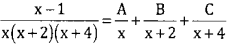Getting Getting A = $$-\frac { 1 }{ 8 }$$ B = $$\frac { 3 }{ 4 }$$ C = $$\frac { -5 }{ 8 }$$
Conclusion

Question 41.
Verify whether the proposition (~p^q)^~r is a tautology or a contradiction or neither.Question 42.
Walking 4 kmph a student reaches his college 5 minutes late and if he walks at 5 kmph he reaches $$2 \frac{1}{2}$$ minutes early. What is the distance from his house to the college?
Let required distance = x kms
At 4 kmph, time taken = $$\frac { x }{ 4 }$$
At 5 kmph, time taken = $$\frac { x }{ 5 }$$
Given $$\frac{x}{4}=\frac{5}{60}=\frac{x}{5}+\frac{2.5}{60} s$$
Getting x = 2.5 kmQuestion 43.
A motor company Ltd. Has observed that a 90° learning effect applies to all labour related costs whenever a new product is taken up for production the anticipated production to 320 units. For the coming year. The at ₹ 15/hour. Calculate the total labour hours and labour cost to manufacture 320 units.
10 units = 1 lot∴ Total hours = 18,895.68
Total labour cost = 18,956.68 × 15 = ₹ 2,83,435.20

Question 44.
Solve the following LPP graphically:
Maximize Z = 60x + 15y
Subjected to the constraints: x + y ≤ 50
3x + y ≤ 90
And x, y ≥ 0Question 45.
If A + B + C = 180°, then prove that sin 2A + sin 2B + sin 2C = 4 sin A sin B sin C.
sin 2A + sin 2B + sin 2C
$$=2 \sin \left(\frac{2 \mathrm{A}+2 \mathrm{B}}{2}\right) \cdot \cos \left(\frac{2 \mathrm{A}-2 \mathrm{B}}{2}\right)+2 \sin \mathrm{C} \cos C$$
= 2 sin C. cos (A – B) + 2 sin C. cos C
= 2 sin C (cos(A – B) + cos C]
= 2 sin C[cos (A – B) + cos {180 – (A + B)}]
= 2 sin C[cos (A – B) – cos (A + B)]
= 2 sin C[-2 sin A . sin(- B)]
= 4 sin A. sin B.sin C

Question 46.
Find the equation of the circle passing through the points (5,3), (1,5) and (3,-1).
Getting 3 equations as
10g + 6f + C = – 34
2g + 10f + C = -26
6g – 2f + C = – 10
Solving to get g = -, f = -2, C = -2
Final equation: x2 + y2 – 4x – 4y – 2 = 0

Question 47.
If y = log$$(x+\sqrt{x^{2}+1})$$ show that (x2 + 1)y2 + xy1 = 0.Question 48.
Find the area bounded by the parabola y2 = 4x and the line x = y.
y2 = 4x … (1)
x = y …. (2)
(1) & (2) ⇒ x = 0, x = 4
Required area
$$=\int_{0}^{4}[f(x)-g(x)] d x$$
$$=\int_{0}^{4}[2 \sqrt{x}-x] d x=\left[2 \times \frac{2}{3} x^{3 / 2}-\frac{x^{2}}{2}\right]_{0}^{4}$$
$$=\frac{32}{3}-\frac{16}{2}=\frac{8}{3}$$ sq.unitsPart – E

Answer any one of the following questions: (1 × 10 = 10)

Question 49.
(a). A sales person Samanth has the following record of sales. He is paid a commission at fixed rate per unit but varying rates for products P, Q and R.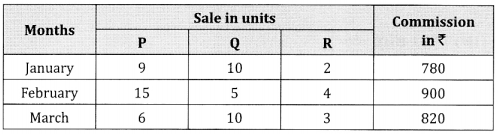Find the rate of commission payable on P, Q and R per unit sold
Let rates of commission per unit of sales be
x,y and z for P, Q and Rrespectively
Writing 9x + 10y + 2z = 780
15x + 5y + 4z = 900
6x + 10y + 3z = 820Note: Proportionate marks should be given to any other appropriate method.

(b). Find the value of (12.)55 using Binomial theorem upto 4 places of decimals.
(1.2)55 = (1 + 0.2)55
= 155 + 5C1(0.2) + 5C2(0.2)2 + 5C3(0.2)3 + 5C4(0.2)4 + (0.2)5
= 1 + 5(0.2) + 10 (0.04) + 10(0.008) + 5(00004) + (0.00032)
= 2.4883Question 50.
(a). If’n’ is a rational number and ‘a’ is a non zero real number, then prove that $$\lim _{x \rightarrow-}\left(\frac{x^{n}-a^{n}}{x-a}\right)=n a^{n-1}$$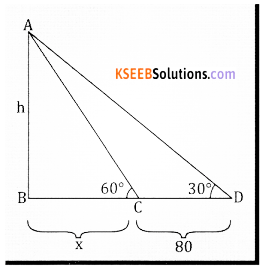tan 60° = $$\frac { h }{ x }$$ ∴ √3 = $$\frac { h }{ x }$$ …. (1)
tan 30° = $$\frac{h}{x+80}$$ ∴ $$\frac{1}{\sqrt{3}}=\frac{h}{x+80}$$ …. (2)